UPSC  >  Solved Examples: Ratio & Proportion

# Solved Examples: Ratio & Proportion - Notes | Study UPSC Prelims Paper 2 CSAT - Quant, Verbal & Decision Making - UPSC

 Table of contentsIntroductionBasics of RatioComposition RatioProportionRatio & ProportionProportions1 Crore+ students have signed up on EduRev. Have you?

Introduction

• If a and b (b ≠ 0) are two quantities of the same kind, then Ratio is the relation which one quantity bears to another of the same kind in magnitude.
• Now in two quantities a and b the fraction a/b is called the ratio of a to b. It is usually expressed as a : b, a and b are said to be the terms of the ratio.
• The former (numerator) ‘a’ is called the Antecedent of the ratio and latter (denominator) ‘b’ is called consequent.
Basics of Ratio
• As ratio is a relation between two quantities so ratio is independent of the concrete units employed in the quantities compared.
• Ratio exists only between two quantities; both the quantities must be in the same units.

Composition Ratio

Compounded Ratio
• When two or more ratios are multiplied term wise, the ratio thus, obtained is called their compounded ratio.
• Compounded ratio of (a : b, c : d and e : f) a/b × c/d × e/f = ace/bdf
Reciprocal Ratio
• For any ratio a : b, the reciprocal ratio will be 1/a : 1/b.
• The application of it is in calculating the ratio of the wages distributed among the workers, which is equal to the reciprocal ratio of number of days taken by them to complete the work.

Proportion

When two ratios are equal, the four terms involved, taken in order are called proportional, and they are said to be in proportion.

• The ratio of a to b is equal to the ratio of c to d i.e. if a/b = c/d, we write a : b : : c : d
• If a : b : : c : d Then we have ad = bc i.e. the terms a and d are called Extremes and, the terms b and c are called the Means.
• The term a, b, c and d are known by the name 1st, 2nd, 3rd and the 4th proportion respectively.
Continued Proportion
• Three quantities are said to be in continued proportion, if the ratio of the first to the second is same as the ratio of the second to the third.
• Thus a, b and c are in continued proportion if a : b = b : c, a/b = b/c
Mean Proportion

If a, b, c are in continued proportion then second quantity ‘b’ is called the mean proportional between ‘a’ and ‘c’ and a, b and c are known as 1st, 2nd and 3rd proportion respectively.

Ratio & Proportion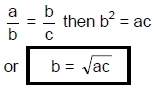Example 1. What is the mean proportional to 5/36, 3.2/25?
(a) 2/25
(b) 15/2
(c) 2/15
(d) 4/25
Ans. (c)
Solution.
Let the mean proportion of 5/36 and 3.2/25 be x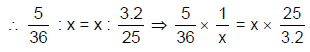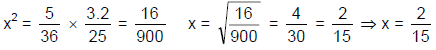Example 2. What should be added to each of the numbers 19, 26, 37, 50 so that the resulting number should be in proportion?
(a) 2
(b) 3
(c) - 2
(d) - 5
Ans. (a)
Solution.
Let the required number be x.
According to question,
19 + x, 26 + x, 37 + x and 50 + x are in proportion
19 + x : 26 + x = 37 + x : 50 + x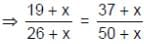(19 + x) (50 + x) = (37 + x) (26 + x)
⇒ x2 + 69x + 950 = x2 + 63x + 962
x2 - x2 + 69x - 63x = 962 - 950
Hence the resulting number = 2.

Basics of Proportion
(i) Let us take four quantities a, b, c & d such that they are in proportion i.e. a : b : : c : d then,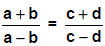This operation is called componendo and Dividendo.

(ii) If a/b = c/d = e/f = then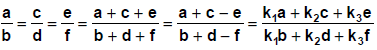Partnership & Share
If there is profit in the business run by two partners A and B then,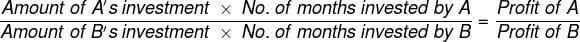Example 3. Saman begins business with a capital of Rs. 50,000 and after 3 months takes Manu into partnership with a capital of Rs. 75000. Three months later Amandeep joined the firm with a capital of Rs. 1, 25,000. At the end of the year the firm makes a profit of Rs. 99,495. How much of this sum should Amandeep receive?
Solution. Money invested by Saman for 12 months = Rs. 50,000
Money invested by Manu for 9 months = Rs. 75000
Money invested by Amandeep for 6 months = Rs. 1,25,000
Share of Saman: Manu: Amandeep
= 50,000 × 12 : 75,000 × 9 ; 1,25,000 × 6
= 6,00,000 : 6,75,000 : 7,50,000 = 600 : 675 : 750 = 8 : 9 : 10
Total profit = Rs. 99,495.
Profit of Amandeep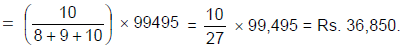Proportions
• Direct Proportion If A is directly proportional to B, then as A increases B also increases proportionally or in other words the proportional change occurs in the same direction.
• In general when A is directly proportional to B, then A/B = Constant
• Examples of Direct proportion are Time & Distance problems; time taken to travel a distance is directly proportional to the distance traveled when the speed is constant.
• This means the distance-traveled doubles if the time taken
doubles provided speed remains constant.

Remember
If x is directly proportional to y,  x1/y1 = x2/y2

Direct Relation
• In these types of cases an increase in one causes increase in the other but the increase is not proportional as in the case of direct proportion.
• For example: With simple interest the amount increases with increase of the number of years but not proportionally while on the other hand interest doubles or triples after 2nd and 3rd years. So the increment in amount is in direct relation while increment in interest is direct proportion.

Remember
If x is in direct relation to y then, x = K1 + yK2

Example 4. If 6 men can lay 8 bricks in one day, then how many men are required to lay 60 bricks in the same time?
(a) 45 men

(b) 40 men
(c) 60 men
(d) 50 men
Ans. (a)
Solution.
Since the time is same so to do more work we need more persons. Hence this is the problem of direct
proportion,
M1/B1 = M2/B2 ⇒ M2 = 6 × 60/8 = 45 men

Example 5. The cost of New Year party organized in TCY is directly related to the number of persons attending that party. If 10 persons attend the party the cost per head is Rs 250 and if 15 people attend, the cost per head is Rs. 200. What will be the total cost of the party if 20 persons attend it?
Solution. This is the problem of direct relation
Let the total cost of party is
Cost = K1 + K2 N (where K1 & K2 are fixed and variable costs and N is number of persons)
250 × 10 = K1 + 10K2 .......... (1)
200 × 15 = K1 + 15K2 .......... (2)
Solving them we get K1 = 1500 and K2 = 100
So total cost for 20 persons = 1500 + 20 × 100 = Rs. 3500

Indirect/Inverse Proportion
• A is in indirect proportion to B if as A increases, B decreases proportionally i.e. the proportional change occurs in the opposite direction.
• In general if A is in indirect proportion to B, then AB = constant.
• Examples of Indirect proportion are Price & Quantity (expenditure remaining same), Number of men required & rate of work done (amount of work remaining same), Time & Speed problems for same distance.

Remeber
If x is inversely proportional to y, then xy = K
⇒ x1y1 = x2y2

Example 6. If 6 men can build a wall in 9 days then 60 men can build a similar wall in ______ days?
Solution. Work = Men x Days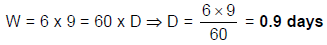Example 7. A can do a piece of work in 12 days, B is 60% more efficient than A. Find the number of days required for B to do the same piece of work.
Solution. Ratio of the efficiencies is A : B = 100 : 160 = 5 : 8. Since efficiency is inversely proportional to the
number of days, the ratio of days taken to complete the job is 8 : 5
So, the number of days taken by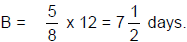Example 8. Ten years ago, the ratio of ages of A and B is 3 : 4, now, it is 4 : 5. What is the present age of A?
Solution. 10 years ago, let their ages be 3k and 4k.
So, present ages are 3k + 10 and 4k + 10.
The ratio is given as 4 : 5.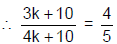k = 10
Percentage of A = 3k + 10 = 40 years.

Example 9. Three friends A, B, C earn Rs. 2000 together. If they want to distribute this money such that ‘A’ should get Rs. 300 more than B and Rs. 100 more than C, in what ratio, they have to distribute the money?
Solution. A = 300 + B = 100 + C
So, A = 300 + B and C = 200 + B
A + B + C = 2000
∴ B = 500 ⇒ A = 800 and C = 700 So, the required ratio = 8 : 5 : 7

Example 10. Four friends A, B, C, D share Rs. 10,500 in the ratio 1/3 : 1/4 : 1/6 : 1/8 how much more money A and C together get than B and D together?
Solution. The given ratio is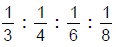Multiply with 24 = 8 : 6 : 4 : 3.
Take A = 8k, B = 6k, C = 4k, D = 3k.
Total = 21k = 10,500
⇒ k = 500
Required answer = (A + C) - (B + D)
= (8k + 4k) - (6k + 3k) = 3k = Rs. 1500

The document Solved Examples: Ratio & Proportion - Notes | Study UPSC Prelims Paper 2 CSAT - Quant, Verbal & Decision Making - UPSC is a part of the UPSC Course UPSC Prelims Paper 2 CSAT - Quant, Verbal & Decision Making.
All you need of UPSC at this link: UPSC

## UPSC Prelims Paper 2 CSAT - Quant, Verbal & Decision Making

67 videos|50 docs|151 tests
 Use Code STAYHOME200 and get INR 200 additional OFF

## UPSC Prelims Paper 2 CSAT - Quant, Verbal & Decision Making

67 videos|50 docs|151 tests

### How to Prepare for UPSC

Read our guide to prepare for UPSC which is created by Toppers & the best Teachers

Track your progress, build streaks, highlight & save important lessons and more!

,

,

,

,

,

,

,

,

,

,

,

,

,

,

,

,

,

,

,

,

,

,

,

,

;